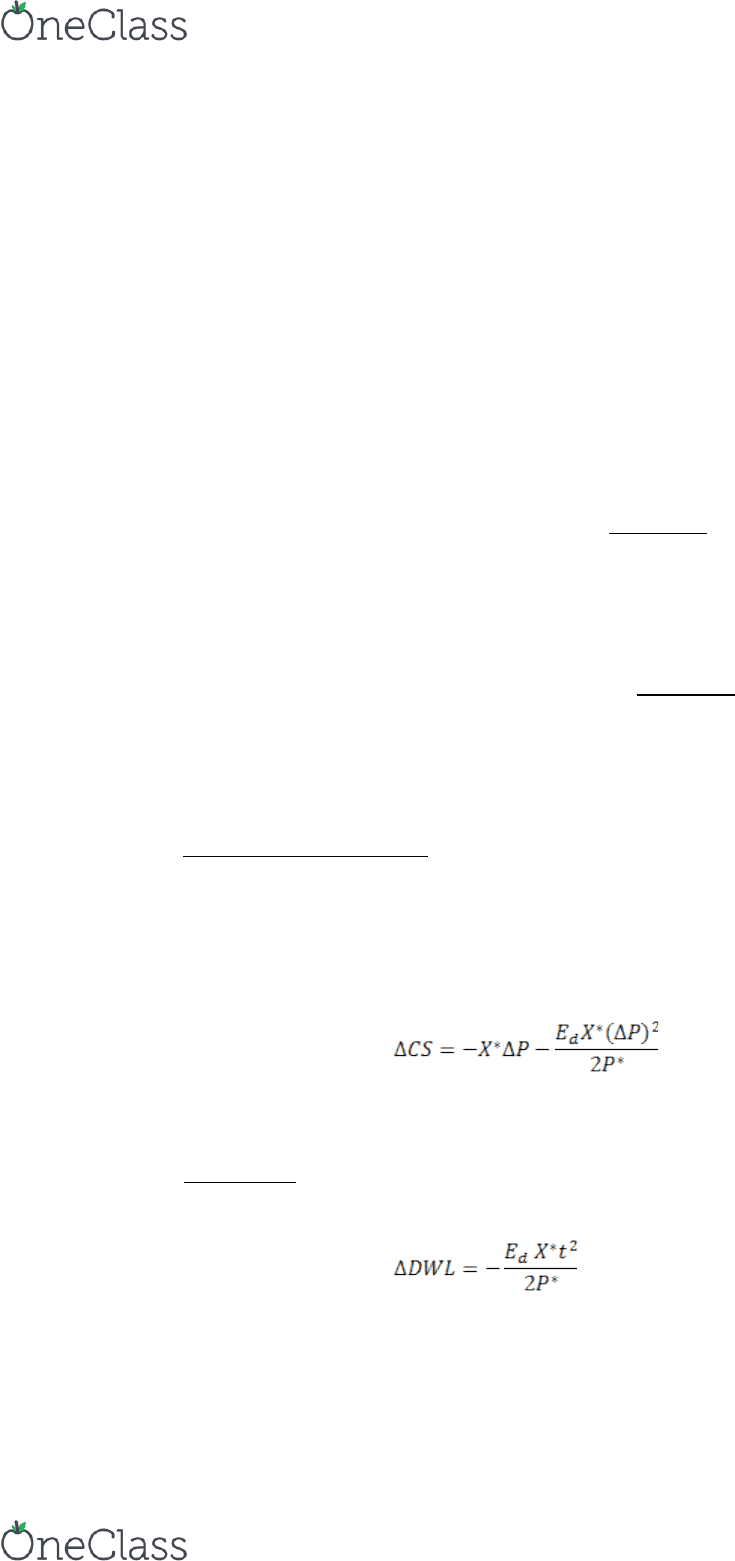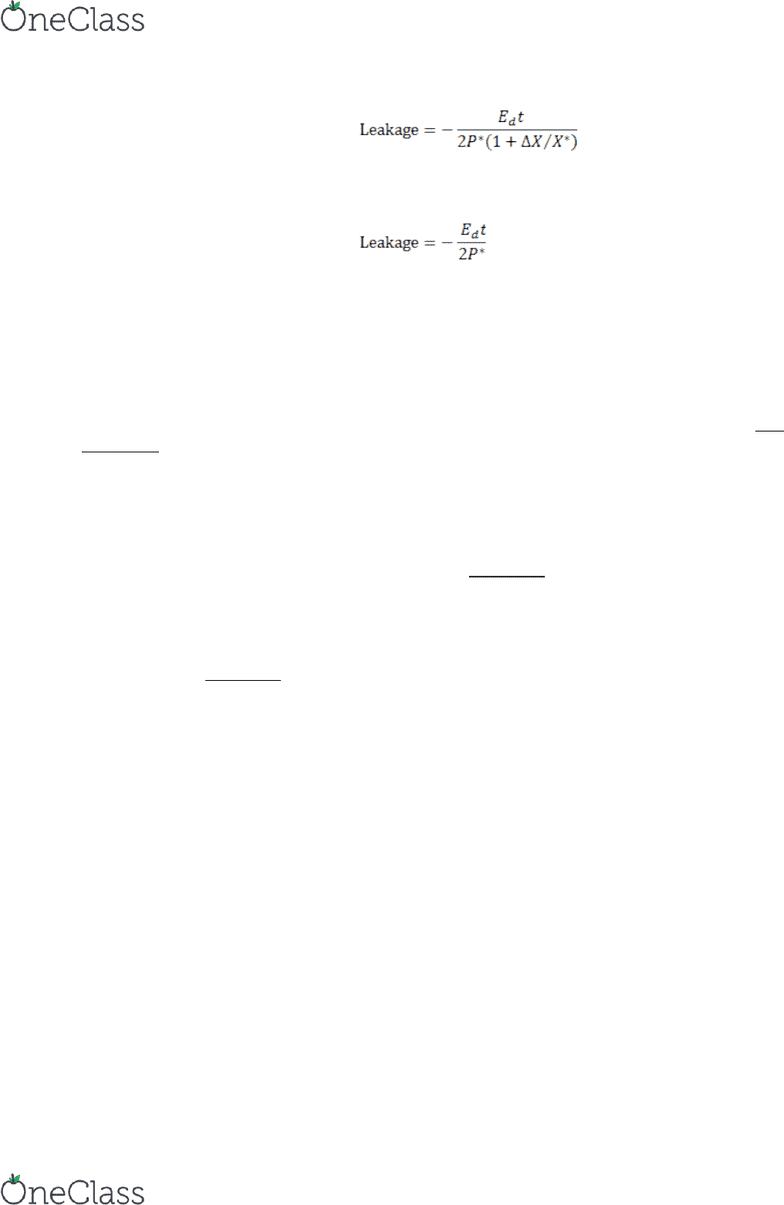# ECON361 Chapter Notes - Chapter 3: Hicksian Demand Function, Budget Constraint, Indifference Curve

83 views5 pages
School
Department
Course
ProfessorCHAPTER 3: ECONOMICS FOUDATIONS OF COST-BENEFIT ANALYSIS
Purpose: To review the microeconomic and welfare economic underpinnings of CBA (assuming
perfect competition).
DEMAND CURVES
Individual demand curves slope down (have a negative slope) due to diminishing marginal utility.
The market demand curve is the horizontal sum of individual demand curves. It also slopes down.
It is important to distinguish between an ordinary demand curve where q = f(p), and an inverse
demand curve in which p = f-1(q). The inverse market demand curve can be interpreted as a
(societal) marginal benefits (MB) curve: it indicates how much (actually the maximum) someone
is willing to pay for an additional unit of a goodthe marginal unit. Consequently the area under
the market demand curve from the origin to X* (see Figure 3.1) measures the gross benefits to
society of consuming X* units of the good.
If one has to pay P* for X* units of the good, then the rectangle bounded by P* and X* is the
aggregate cost to consumers. The net benefits to consumers are the gross benefits minus the
consumers’ costs. The net benefits are called the consumer surplus (CS): it is the area between the
demand curve and the P* line—the light shaded area in Figure 3.1. Consumer surplus is
important in CBA because changes in CS can be viewed as close approximations of the WTP for
(the benefits of) a policy change.
Changes in Consumer Surplus
Figures Figures 3.2 (a) and 3.2(b) show the changes in CS due to a price decrease or increase,
respectively. If the demand curve is linear, the change in CS is given by equation 3.2. This is a
good approximation even if the demand curve is not linear.
Sometimes the demand curve is not linear but the analyst knows the price elasticity of demand. In
this case the change in CS may be computed using equation 3.4:
Taxes are important in CBA because governments often raise funds for government projects
through taxes. Unfortunately, taxes result in a reduction in CS, represented by the triangular area
ABC in Figure 3-2b. This is an example of deadweight loss. The deadweight loss due to a tax is
called the deadweight loss of taxation. For a unit tax, like an excise tax, it can be computed using
equation 3.6:
Leakage is the ratio of the deadweight loss to the total tax revenue raised; see equations 3.7 and
3.8.
Boardman, Greenberg, Vining, Weimer / Cost-Benefit Analysis, 4th Edition
Instructor's Manual 3-1
find more resources at oneclass.com
find more resources at oneclass.com
Unlock document

This preview shows pages 1-2 of the document.
Unlock all 5 pages and 3 million more documents.

Already have an account? Log in(3.7)
If the change in output is relatively small, then the following simple formula provides a very slight
over-estimate of the leakage:
(3.8)
SUPPLY CURVES
The upward sloping segment of a firm’s marginal cost curve above its average variable cost curve
is its supply curve (below the average variable cost, the firm would shut down). The marginal
cost curve indicates the additional cost to produce each additional unit of the good. The area
under the curve represents the total variable cost of producing a given amount of the good; see
Figure 3-3. To an economist all costs are opportunity costs (i.e., they should reflect the value of
goods and services in their best alternative use). One should include the opportunity cost of
capital and entrepreneurship.
Producer surplus is the supply-side equivalent of consumer surplus. It is the difference between
total revenues (a rectangle bounded by P* and X*) and total variable costs. Diagrammatically, it is
the area between the price and the supply curve; see Figure 3.4.
SOCIAL SURPLUS AND ALLOCATIVE EFFICIENCY
Ignoring government surplus, consumer surplus plus the producer surplus equals social surplus.
Graphically (see Figure 3.5), social surplus is the area between the demand and supply curves to
the left of the equilibrium point. Since demand reflects MB and supply reflects MC, net social
benefits (social surplus) is maximised where the supply and demand curves intersect. Since this
equilibrium price (P*) and output level (X*) come about under perfect competition, we see that
perfect competition maximises social surplus. This point is a Pareto optimum point and it is
allocatively efficient.Note also P = MC that at that point
Any policy intervention by government in a perfectly competitive market reduces social surplus
and creates a deadweight loss.
Profits and Factor Surplus
Thus far we have implicitly focussed on short-run changes, assuming that some inputs are fixed.
However, in practice, CBA projects often involve changes in fixed costs which should be taken
into account. Also, it is possible that producer surplus is captured by some particular factors of
production, such as from a particularly productive plot of land. Such surplus, which are a form of
Ricardian rents, is called factor surplus (FS). One way to deal with these two issues is to
recognise that PS = π +FS, where π = economic profits. Consequently, social surplus and changes
in social surplus can be obtained from equation 3.11:
SS = CS + π + FS (3.11a)
ΔSS = ΔCS +Δπ + ΔFS (3.11b)
Boardman, Greenberg, Vining, Weimer / Cost-Benefit Analysis, 4th Edition
Instructor's Manual 3-2
find more resources at oneclass.com
find more resources at oneclass.com
Unlock document

This preview shows pages 1-2 of the document.
Unlock all 5 pages and 3 million more documents.

Already have an account? Log in

# Get access

Grade+
\$10 USD/m
Billed \$120 USD annually
Homework Help
Class Notes
Textbook Notes
40 Verified Answers
Study Guides
1 Booster Class
Class+
\$8 USD/m
Billed \$96 USD annually
Homework Help
Class Notes
Textbook Notes
30 Verified Answers
Study Guides
1 Booster Class